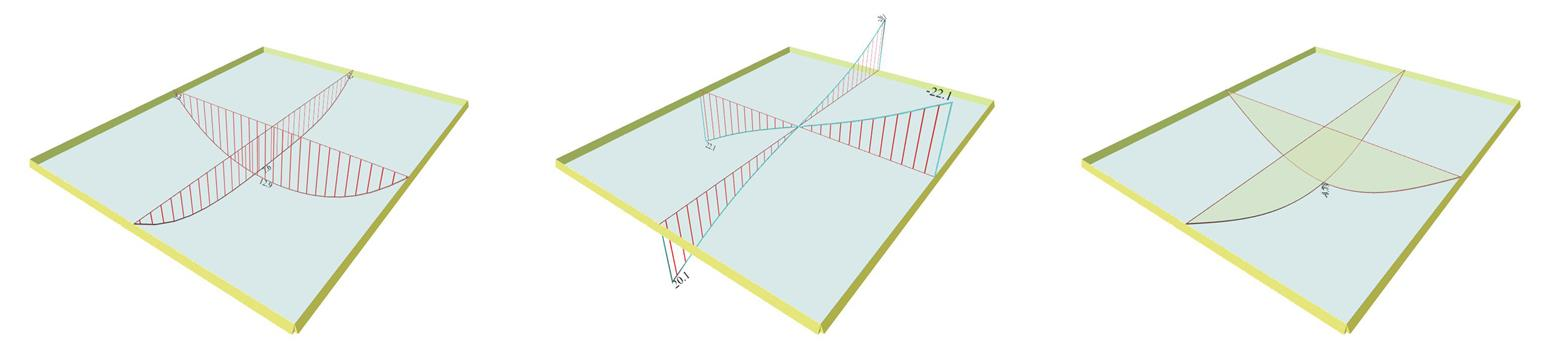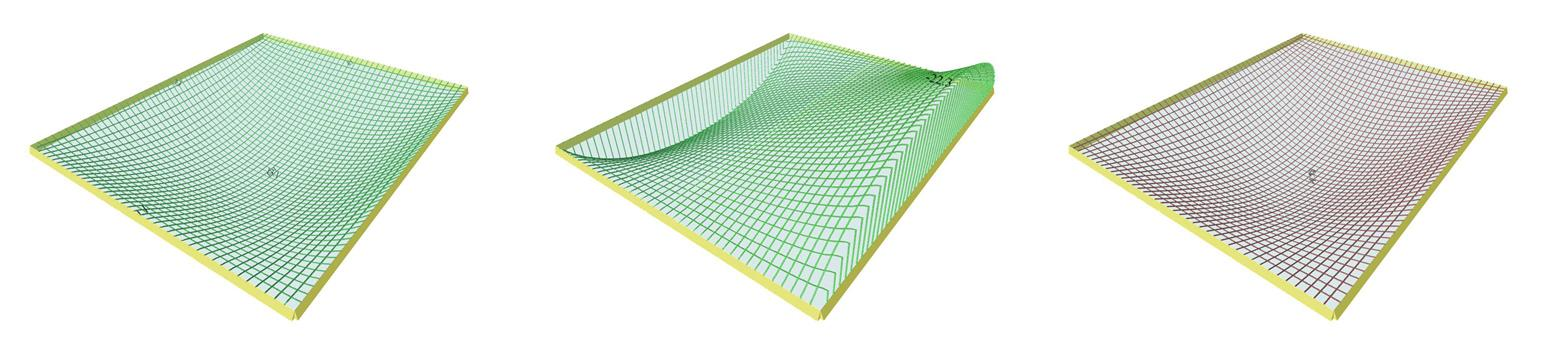« Slabs supported on three or two adjacent edges Exercise 4.9.2 »

Exercises

Exercise 4.9.1

Given: Covering load ge=1.00 kN/m2, live load q=5.00 kN/m2, concrete quality C30/37 (E=32.8 GPa).

Question : Perform static analysis to determine bending moments, shear forces, support reaction forces and elastic deflection.

Solution:

Self-weight: go=0.17m·25.0kN/m3= 4.25 kN/m2

Total live load: q= 5.00 kN/m2

The design combination in ultimate limit state (ULS) is obtained by load p=γg·g+γ q·q equal to:

p=1.35g+1.50q=1.35x5.25+1.50x5.00=14.59 kN/m2

Three different methods for the calculation of the two-way slab, by means of Marcus, Czerny and finite element method are presented below.

Marcus method:

lx= 4.00 m,ly=5.00 mε=ly/lx=5.00/4.00=1.25

Table b5.1 for ε=1.25 gives:

Bending moments :

kx=0.709 , ky=0.291, vx=vy=0.622

px=kx·p·1.0m=0.709x14.59kN/m2x1.0m=10.34 kN/m

py=ky·p·1.0m=0.291x14.59kN/m2x1.0m=4.25 kN/m

Mx=vx·px · lx2/8=0.622x10.34x(4.02/8)=12.86 kNm

My=vy·py · ly2/8=0.622x4.25x5.02/8=8.26 kNm

Shear forces :

Deflection:

am=0.0689fm=am·p·lx4/(E·h3)= 0.0689x14.59x103N/m2x4.04m4/(32.80x109N/m2x1.0mx0.173m3)

fm =1597x10-6m=1.597 mm

Equivalent uniform reaction forces :

υxr=0.300, υyr=0.250

Czerny method:

Table 46, for ε=1.25 gives:

Bending moments:

mx=17.80 , my=29.90

Mx=p·lx2/mx=14.59x4.002/17.80=13.11 kNm

My=p·lx2/my=14.59x4.02/29.90=7.81 kNm

Shear forces :

ρxr=0.39, ρyr=0.36

Vxrxr·p·lx=0.39x14.59kN/m2x4.00m=22.76 kN/m

Vyryr·p·ly=0.36x14.59kN/m2x4.00m=21.01 kN/m

Deflection:

100a=7.28a=0.0728

fm=a·p·lx4/(E·h3)=0.0728x14.59x103N/m2x4.04m4/(32.80x109N/m2x0.173m 3)=1.687 mm

Equivalent uniform reaction forces:

Equivalent uniform reaction forces are determined in exactly the same way as in Marcus method.

Finite element method:

In project < B_49-1>, the following results are obtained by activating module "SLABS" in pi-FES:Figure 4.9.1-2: Results summary from "Slab Results" module in pi-FES Figure 4.9.1-2: Results summary from "Slab Results" module in pi-FES Mx=12.9 kNm, My=7.6 kNm Vx=22.1 kN, Vy=20.1 kN y=-1.73 mmFigure 4.9.1-3: Analytical results from "FEM Results" of pi-FES Figure 4.9.1-3: Analytical results from "FEM Results" of pi-FES Bending moment distribution M11 Shear force distribution V11 Deflections

Equivalent uniform reaction forces are determined in exactly the same way as in Marcus method.

Summarizing,

• Marcus method gives:

Mx / My=12.86 / 8.26 kNm

Vxr / Vyr=29.18 / 29.18 kN

f=1.60 mm

Mx / My=13.11 / 7.81 kNm

Vxr / Vyr=22.76 / 21.01 kN

f=1.69 mm

• Finite element method gives:

Mx/My=12.9 / 7.6 kNm

Vxr/Vyr=22.1 / 20.1 kN

f=1.73 mm

Notes :

« Slabs supported on three or two adjacent edges Exercise 4.9.2 »